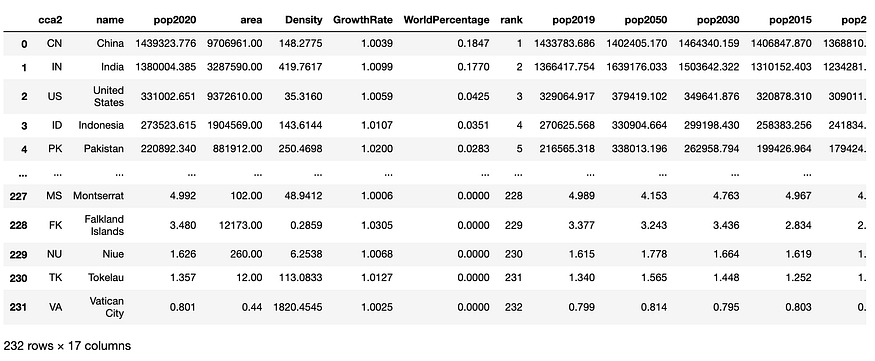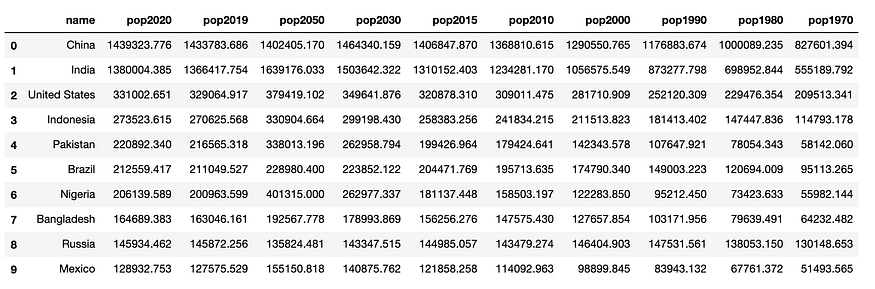# Filtering Rows and Columns in Pandas Python — techniques you must know

In this tutorial, I will focus on the most commonly used techniques to filter and subset data for data frames, which will be widely applied in your future data analysis projects.The tutorial is based on the real data which can be downloaded from the website worldpopulationreview.

### Import libraries and data

``````import pandas as pd
dataset
``````

You get a dataset with 232 rows and 17 columns like thisIf you would like to know how to get the data without using importing, you can read my other post — Make Beautiful Nightingale Rose Chart in Python.

#### Filtering rows based by conditions

1. Filtering rows on meeting one condition

The syntax of filtering row by one condition is very simple — dataframe[condition].

``````#Showing data of Brazil only
dataset[dataset['name']=='Brazil']  #Method 1
``````

In Python, the equal operator is ==, double equal sign.

Another way of achieving the same result is using Pandas chaining operation. It is very convenient to use Pandas chaining to combine one Pandas command with another Pandas command or user defined functions. Here we can use Pandas eq() function and chain it with the name series for checking element-wise equality to filter the data.

``````dataset[dataset.name.eq('Brazil')]  #Method 2
``````

The syntax of filtering row by more than one conditions is dataframe[(condition1) & (condition2)]

``````#Showing only the rows in which the pop2020 is more than 50,000 AND
Density is higher than 100:
dataset[(dataset['pop2020'] > 50000) & (dataset['Density'] > 100)]
``````

3. Filtering rows on NOT meeting one condition

In Python, the equal operator is !=, exclamation sign followed by an equal sign.

``````#Excluding China from the data
dataset[dataset['name']!='China']. #Method 1

dataset[dataset.name!='China'] #Method 2
``````

4. Filtering rows on NOT meeting more than one conditions

``````dataset[(dataset['name']!='China') & (dataset['name']!='India')] Method 1
dataset[(dataset.name!='China') & (dataset.name!='India')] # Method 2
``````
1. Filtering rows based on a list

What if we would like to get data of 20 countries we are interested in for further analysis? Write code using above-mentioned method like dataframe[(condition1) & (condition2) & ….&[condition20)] is very inefficient. We need to use isin() function to solve this problem. It allows us to select rows using a list or any iterable.

We need to create a list consisting names of 20 countries and then pass the list to isin() function.

``````Listname = ['France', 'Italy', 'South Africa', 'Tanzania', 'Myanmar', 'Kenya','South Korea', 'Colombia', 'Spain', 'Argentina', 'Uganda','Ukraine', 'Algeria', 'Sudan', 'Iraq', 'Afghanistan', 'Poland','Canada', 'Morocco', 'Saudi Arabia']
dataset[dataset.name.isin(Listname)]
``````

Trick: you don't need to write country names one by one. You can use dataset.name.unique() to get unique country names, then copy and paste names you would like to select.

1. Filtering rows based on values NOT in a list
``````Listname_excl = ['China', 'India', 'United States', 'Indonesia', 'Pakistan']
dataset[~dataset.name.isin(Listname_excl)]
``````
1. Filtering rows containing certain characters
``````# select rows containing 'Korea'
dataset[dataset.name.str.contains(“Korea”)]
``````
1. Filtering rows based on row number
``````dataset.filter(regex='0\$', axis=0) #select row numbers ended with 0, like 0, 10, 20,30
``````

### Filtering columns based by conditions

1. Filtering columns containing a string or a substring

If we would like to get all columns with population data, we can write

``````dataset.filter(like = 'pop', axis = 1). #Method 1
``````

In the bracket, like will search for all columns names containing 'pop'. The 'pop' doesn't need to be the starting of the column names. If we put like='n', we will get data from columns — 'name', 'Density', 'WorldPercentage', and 'rank' because they all have 'n' in their names.

We can also use regex to get the same result.

``````dataset.filter(regex = 'pop', axis = 1). #Method 2
``````

If you would like to select column names starting with pop, just put a hat ^pop.

Another way of filtering the columns is using loc and str.contains() function.

``````dataset.loc[:, dataset.columns.str.contains('pop')] #Method3
``````

Those three methods will give you the same result but personally, I recommend the second method. It give you more flexibility while less coding required.

1. Filtering columns containing more than one different strings

If we would like to get the country and all population data, we need to use |, which is the logical OR operator in regex.

``````dataset.filter(regex = '^pop|name', axis = 1)
``````

You will get a nice dataset in good order like this:## Removing rows with missing data

dropna() function will drop the rows where at least one element is missing.

``````dataset.dropna(axis=0)
``````

If you want to drop the rows where all elements are missing.

``````df.dropna(how='all')
``````

Now, you are able to filter and subset dataset according to your own requirements and needs. Congratulations!

If you would like to learn how to slice data in Pandas, please don't miss my other post Slicing data in Pandas Python — techniques you must know.